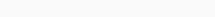# Math Puzzles Puzzle 21 Answer AndroidMath puzzle game level 21 solution with detailed hints and explanation, Scroll below to find out.

Math puzzles increase your logical thinking. The objective of the game is to find patterns hidden in the puzzle in order to arrive at the solution. Challenge yourself with different levels of math puzzles and stretch the limits of your intelligence.

### Math Puzzles Puzzle 21:

How many triangles are there?Solution/Hint: Count the number of triangles

9 small triangles are easy to see

when you combine upper 4 triangles, you will another triangle of 2*2*2 size, thesetrianglese are 3 in 3 corners.

And one big triangle.

total-9+3+1=13

If there is any Doubt or you are unable to understand the solution, then please let me know in comments, I will surely help you.